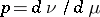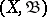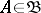##### Actions

A chargethat is absolutely continuous with respect to some measurehas a densitywith respect tothat is summable with respect to this measure. It was established by J. Radon  and O.M. Nikodým . More precisely, on a measurable space, whereis a-algebra of subsets of, suppose one is given a charge, i.e. a countably-additive real or complex function given on, and a-finite measure, and, moreover, letbe absolutely continuous with respect to. Then there is a function,, summable with respect to, such that for any set,The functionis unique (except for modifications on a set of-measure zero), and is called the density of the chargewith respect to the measure. There are (see ) generalizations of the theorem to the case when the charge takes values in some vector space.